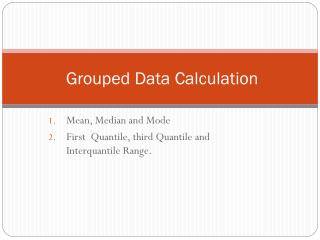DownloadDownload PresentationGrouped Data Calculation

# Grouped Data Calculation

Download Presentation## Grouped Data Calculation

- - - - - - - - - - - - - - - - - - - - - - - - - - - E N D - - - - - - - - - - - - - - - - - - - - - - - - - - -
##### Presentation Transcript

1. Grouped Data Calculation Mean, Median and Mode First Quantile, third Quantile and Interquantile Range.

2. Measure of the Central Tendency

3. Mean – Grouped Data • Themean may often be confused with the median, mode or range. The mean is the arithmetic average of a set of values, or distribution. Example: The following table gives the frequency distribution of the number of orders received each day during the past 50 days at the office of a mail-order company. Calculate the mean. Solution: X is the midpoint of the class. It is adding the class limits and divide by 2.

4. Median and Interquartile Range– Grouped Data • amedian is described as the numerical value separating the higher half of a sample, a population, or a probability distribution, • Step 1: Construct the cumulative frequency distribution. • Step 2: Decide the class that contain the median. • Class Median is the first class with the value of cumulative • frequency equal at least n/2. • Step 3: Find the median by using the following formula: Where: n = the total frequency F = the cumulative frequencybefore class median = the frequency of the class median i = the class width = the lower boundary of the class median

5. Example: Based on the grouped data below, find the median: Solution: 1st Step: Construct the cumulative frequency distribution class median is the 3rd class So, F = 22, = 12, = 20.5 and i = 10

6. Therefore, Thus, 25 persons take less than 24 minutes to travel to work and another 25 persons take more than 24 minutes to travel to work.

7. Quartiles • a quartile is one of three points that divide a data set into four equal groups, each representing a fourth of the distributed sampled population. • Using the same method of calculation as in the Median, • we can get Q1 and Q3 equation as follows: Example: Based on the grouped data below, find the Interquartile Range

8. Solution: 1st Step: Construct the cumulative frequency distribution 2nd Step: Determine the Q1 and Q3 Class Q1 is the 2nd class Therefore,

9. Class Q3 is the 4th class Therefore, Interquartile Range IQR = Q3 – Q1 IQR = Q3 – Q1 calculate the IQ IQR = Q3 – Q1 = 34.3889 – 13.7143 = 20.6746

10. Mode – Grouped Data • Mode • Mode is the value that has the highest frequency in a data set. • For grouped data, class mode (or, modal class) is the class with the highest frequency. • To find mode for grouped data, use the following formula: Where: i is the class width is the difference between the frequency of class mode and the frequency of the class after the class mode is the difference between the frequency of class mode and the frequency of the class before the class mode is the lower boundary of class mode

11. Calculation of Grouped Data - Mode Example: Based on the grouped data below, find the mode Solution: Based on the table, = 10.5, = (14 – 8) = 6, = (14 – 12) = 2 and i = 10

12. Variance and Standard Deviation-Grouped Data Population Variance: Variance for sample data: Standard Deviation: Population: Sample: •  the variance is used as a measure of how far a set of numbers are spread out from each other. • Standard deviation is a widely used measurement of variability or diversity used in statistics and probability theory. It shows how much variation or "dispersion" there is from the average (mean, or expected value).

13. Example: Find the variance and standard deviation for the following data: Solution:

14. Variance, Standard Deviation, Thus, the standard deviation of the number of orders received at the office of this mail-order company during the past 50 days is 2.75.